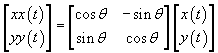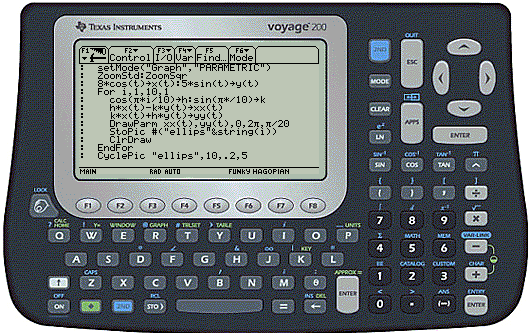# Creating an Animated Rotation on the TI Voyage 200 Calculator

The program below can be used to create the animation shown here on the TI Voyage 200 or the TI89.  When you enter and execute this program on either a V200 or TI89 calculator, you will see the animation shown here.``` rot() Prgm   setMode("Graph","PARAMETRIC")   ZoomStd:ZoomSqr   //Set initial parametric function--ellipse centered at origin   8*cos(t)->x(t)      3*sin(t)->y(t)   //Create 10 images, each rotated 18 degrees counterclockwise   //since 10*0.314 is approximately pi, it will be one complete rotation   //Name the rotated functions something different   //(xx(t), instead of x(t) here)   //to avoid feedback loop leading to memory errors.   For i,1,10,1     // Compute the rotation matrix parameters     cos(.314*i)->h: sin(.314*i)->k     // Rotate.     h*x(t)-k*y(t)->xx(t)     k*x(t)+h*y(t)->yy(t)     //Draw the rotated parametric equations.     DrawParm xx(t),yy(t),0,6.3,015     //Store the pictures     StoPic #("ellips"&string(i))     ClrDraw   EndFor   // Cycle the 10 pictures at .2sec/pic, 5 times   CyclPic "ellips",10,.2,5 EndPrgm     ```